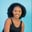Related Tags

pandas
python3
python

# How to use the Series.expanding() function in pandasMaria Elijah

## Overview

The Series.expanding() function in the pandas library in Python lets us perform an expanding windows calculation over data in the given series.

### Syntax

Series.expanding(min_periods=1, center=None, axis=0)


### Parameters:

• min_periods: This represents the minimum number of observations needed to have a value in the window. The default value is None.

• center: This sets the labels of the window. The default is False. The window labels are set to the right edge of the window index if the center value is False. Otherwise, the window labels are set at the center of the window index.

• axis: This is an int or string. The default value is 0. If the axis is set to 0 or index, the operation is performed across the rows. If the axis is set to 1 or columns, the operation is performed across the columns.

### Code example

The following code demonstrates how to use the Series.expanding() function in pandas:

import pandas as pd

# Create the series
series=pd.Series(range(1,27,3), index=[x for x in 'educative'])

# Print the series
print(series)

# Return the expanding transformation of the series
# using Series.expanding()
print("Result of Series.expanding()")
print(series.expanding(2).sum())

### Code explanation

In the code above:

• Line 1: We import the pandas library.

• Line 5: We create a series using the range() function and set the index to educative.

• Line 7: We display the series elements.

• Lines 10–11: We calculate the expanding sum of data in the series over two observations using the expanding() function.

Note: Since there is no element preceding the first element in the series, the sum operation could not be performed on the first element. Hence, NaN is returned.

RELATED TAGS

pandas
python3
python

CONTRIBUTORMaria Elijah
RELATED COURSES

View all Courses

Keep Exploring

Learn in-demand tech skills in half the time